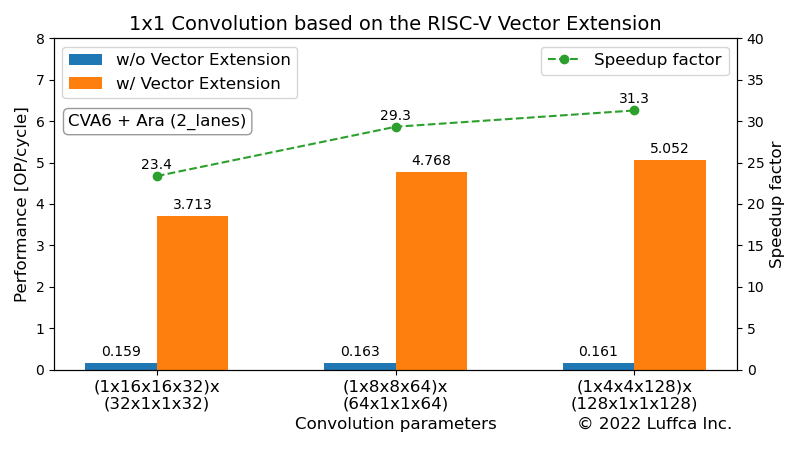# 1×1 Convolution based on the RISC-V Vector ExtensionWe have created a 1×1 convolution kernel based on the RISC-V Vector Extension (RVV) and evaluated its performance using an RTL simulator.

## 1×1 Convolution

A 1×1 convolution computes the dot product of each (h, w) input of size HxWxC with a filter of size 1x1xC for each output channel.

The pre-vectorized code with reference to TensorFlow Lite for Microcontrollers looks like this:

```// output_data = 1x1_conv((input_data + input_offset), filter_data) with
// input_data = [B, H, W, C], filter_data = [OC, 1, 1, C],
// output_data = [B, H, W, OC]
void OneByOneConvInt8(const int8_t* input_data, const int8_t* filter_data,
int32_t* output_data, const int32_t input_offset, ...) {
...
for (int batch = 0; batch < batches; ++batch) {
for (int out_y = 0; out_y < output_height; ++out_y) {
const int in_y = out_y;
for (int out_x = 0; out_x < output_width; ++out_x) {
const int in_x = out_x;
for (int out_channel = 0; out_channel < output_depth; ++out_channel) {
int32_t acc = 0;
for (int in_channel = 0; in_channel < input_depth; ++in_channel) {
int32_t input_val =
input_data[Offset(input_shape, batch, in_y, in_x, in_channel)];
int32_t filter_val = filter_data[Offset(filter_shape, out_channel,
0, 0, in_channel)];
acc += (input_val + input_offset) * filter_val;
}
output_data[Offset(output_shape, batch, out_y, out_x, out_channel)] =
acc;
}
}
}
}
}
```

Note that input_data and filter_data are `int8_t` type arrays, and output_data is `int32_t` type arrays.

## Ara

Ara is an implementation of the RISC-V Vector Extension developed by the Parallel Ultra Low Power (PULP) project. For an overview of Ara, see the related article Matrix Multiplication based on the RISC-V Vector Extension.

## 1x1 Convolution on Ara RTL Simulator

Ara can create an RTL simulator for Ara system combining CVA6 and Ara, using Verilator. This time, we used the minimal configuration `2_lanes.mk` with two 64-bit vector units.

Below is the console output when running the 1x1 convolution kernel.

```\$ cd \$ARA/hardware
\$ app=1x1_conv_int8 make simv

...

===================
=  1X1 CONV INT8  =
===================

--------------------------------------------------------------------
Calculating a (1 x 16 x 16 x 32) x (32 x 1 x 1 x 32) convolution...
--------------------------------------------------------------------

Initializing data...
Calculating 1x1 conv without vector extension...
The execution took 3300106 cycles.
The performance is 0.159 OP/cycle.
Calculating 1x1 conv with vector extension...
The execution took 141214 cycles.
The performance is 3.713 OP/cycle.
Verifying result...
Passed.

--------------------------------------------------------------------
Calculating a (1 x 8 x 8 x 64) x (64 x 1 x 1 x 64) convolution...
--------------------------------------------------------------------

Initializing data...
Calculating 1x1 conv without vector extension...
The execution took 3224180 cycles.
The performance is 0.163 OP/cycle.
Calculating 1x1 conv with vector extension...
The execution took 109951 cycles.
The performance is 4.768 OP/cycle.
Verifying result...
Passed.

--------------------------------------------------------------------
Calculating a (1 x 4 x 4 x 128) x (128 x 1 x 1 x 128) convolution...
--------------------------------------------------------------------

Initializing data...
Calculating 1x1 conv without vector extension...
The execution took 3247290 cycles.
The performance is 0.161 OP/cycle.
Calculating 1x1 conv with vector extension...
The execution took 103775 cycles.
The performance is 5.052 OP/cycle.
Verifying result...
Passed.
```

For 32, 64 and 128 input/output channels, we have achieved a speedup of 23-31 times over CVA6.

## Summary

We created a 1x1 convolution kernel based on the RISC-V Vector Extension and evaluated its performance using Ara's RTL simulator.

Previous article

Next article